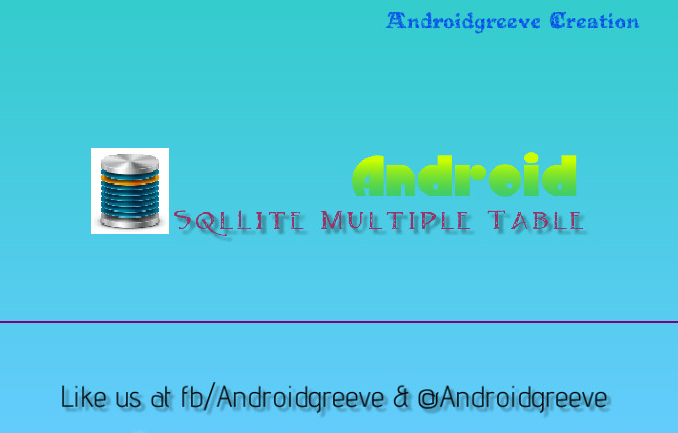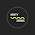# Android SQLite Multiple table basics

In the previous  post we created Sqllite Database with Single table in it.But a database can contain multiple table .So, in this tutorial we will be Looking How to create multiple tables in single database as"Android Sqlite Basics for Multiple tables ".lets start by creating three simple tables

So let’s start by creating a new project in Eclipse IDE

1. Create a new project in Eclipse from File ? New ? Android ? Application Project. I named my package name as info.androidgreeve.sqlite and left the main activity name as MainActivity.java

2. We need two more packages to keep helpers and model classes. Right Clicking on src ? New ? Package and name them as info.androidgreeve.sqlite.helper and info.androidgreeve.sqlite.model

Creating Model Class for Tables

Next step is to create model classes for our database tables just to make single row as an object. We need only two models for Tasks and Cat. For task_cat we don’t need a model class.

3. Create a new class file under info.androidgreeve.sqlite.helper package named Tasks.java and type the code like below. This is the model class for Tasks table

package info.androidgreeve.sqlite.model;

int id;
String note;
int status;
String created_at;

// constructors
}

public Task(String note, int status) {
this.note = note;
this.status = status;
}

public Task(int id, String note, int status) {
this.id = id;
this.note = note;
this.status = status;
}

// setters
public void setId(int id) {
this.id = id;
}

public void setNote(String note) {
this.note = note;
}

public void setStatus(int status) {
this.status = status;
}

public void setCreatedAt(String created_at){
this.created_at = created_at;
}

// getters
public long getId() {
return this.id;
}

public String getNote() {
return this.note;
}

public int getStatus() {
return this.status;
}
}

4. Create one more model class for Cat table named Cat.java under the same package.

cat.java

package info.androidgreeve.sqlite.model;

public class cat {

int id;
String cat_name;

// constructors
public cat() {

}

public cat(String cat_name) {
this.cat_name = cat_name;
}

public cat(int id, String cat_name) {
this.id = id;
this.cat_name = cat_name;
}

// setter
public void setId(int id) {
this.id = id;
}

public void setcatName(String cat_name) {
this.cat_name = cat_name;
}

// getter
public int getId() {
return this.id;
}

public String getcatName() {
return this.cat_name;
}
}

Database Helper Class

Database helper class contains all the methods to perform database operations like opening connection, closing connection, insert, update, read, delete and other things. As this class is helper class, place this under helper package.
So create another class named DatabaseHelper.java under info.androidgreeve.sqlite.helper package and extend the class from SQLiteOpenHelper

public class DatabaseHelper extends SQLiteOpenHelper {

6. Add required variables like database name, database version, column names. I also executed table create statements in onCreate() method. Type the following code in DatabaseHelper.java class

DatabaseHelper.java

public class DatabaseHelper extends SQLiteOpenHelper {

// Logcat cat
private static final String LOG = "DatabaseHelper";

// Database Version
private static final int DATABASE_VERSION = 1;

// Database Name
private static final String DATABASE_NAME = "contactsManager";

// Table Names
private static final String TABLE_cat = "cats";

// Common column names
private static final String KEY_ID = "id";
private static final String KEY_CREATED_AT = "created_at";

// NOTES Table - column nmaes
private static final String KEY_STATUS = "status";

// catS Table - column names
private static final String KEY_cat_NAME = "cat_name";

// NOTE_catS Table - column names
private static final String KEY_cat_ID = "cat_id";

// Table Create Statements
private static final String CREATE_TABLE_tasks = "CREATE TABLE "
+ TABLE_tasks + "(" + KEY_ID + " INTEGER PRIMARY KEY," + KEY_tasks
+ " TEXT," + KEY_STATUS + " INTEGER," + KEY_CREATED_AT
+ " DATETIME" + ")";

// cat table create statement
private static final String CREATE_TABLE_cat = "CREATE TABLE " + TABLE_cat
+ "(" + KEY_ID + " INTEGER PRIMARY KEY," + KEY_cat_NAME + " TEXT,"
+ KEY_CREATED_AT + " DATETIME" + ")";

private static final String CREATE_TABLE_tasks_cat = "CREATE TABLE "
+ TABLE_tasks_cat + "(" + KEY_ID + " INTEGER PRIMARY KEY,"
+ KEY_tasks_ID + " INTEGER," + KEY_cat_ID + " INTEGER,"
+ KEY_CREATED_AT + " DATETIME" + ")";

public DatabaseHelper(Context context) {
super(context, DATABASE_NAME, null, DATABASE_VERSION);
}

@Override
public void onCreate(SQLiteDatabase db) {

// creating required tables
db.execSQL(CREATE_TABLE_cat);
}

@Override
public void onUpgrade(SQLiteDatabase db, int oldVersion, int newVersion) {
// on upgrade drop older tables
db.execSQL("DROP TABLE IF EXISTS " + TABLE_tasks);
db.execSQL("DROP TABLE IF EXISTS " + TABLE_cat);
db.execSQL("DROP TABLE IF EXISTS " + TABLE_tasks_cat);

// create new tables
onCreate(db);
}

Create, Read, Update and Delete) Operations

From now on we are going to add one by one method into DatabaseHelper.class

The function will create a task item in tasks table. In this same function we are assigning the task to a tag name which inserts a row in task_cat table.

SQLiteDatabase db = this.getWritableDatabase();

ContentValues values = new ContentValues();
values.put(KEY_CREATED_AT, getDateTime());

// insert row

for (long cat_id : cat_ids) {
}

}

SELECT * FROM TASKS WHERE id = 1;

String selectQuery = "SELECT  * FROM " + TABLE_TASK + " WHERE "
+ KEY_ID + " = " + TASK_id;

Log.e(LOG, selectQuery);

Cursor c = db.rawQuery(selectQuery, null);

if (c != null)
c.moveToFirst();

td.setId(c.getInt(c.getColumnIndex(KEY_ID)));
td.setCreatedAt(c.getString(c.getColumnIndex(KEY_CREATED_AT)));

return td;
}

String selectQuery = "SELECT  * FROM " + TABLE_task;

Log.e(LOG, selectQuery);

Cursor c = db.rawQuery(selectQuery, null);

// looping through all rows and adding to list
if (c.moveToFirst()) {
do {
td.setId(c.getInt((c.getColumnIndex(KEY_ID))));
td.setCreatedAt(c.getString(c.getColumnIndex(KEY_CREATED_AT)));

} while (c.moveToNext());
}

}

4. Fetching all Task under a cat name

SELECT * FROM tasks td, cats tg, task_cats tt WHERE tg.cat_name = ‘Manner’ AND tg.id = tt.cat_id AND td.id = tt.task_id;

String selectQuery = "SELECT  * FROM " + TABLE_task + " td, "
+ TABLE_cat + " tg, " + TABLE_task_cat + " tt WHERE tg."
+ KEY_cat_NAME + " = '" + cat_name + "'" + " AND tg." + KEY_ID
+ " = " + "tt." + KEY_cat_ID + " AND td." + KEY_ID + " = "

Log.e(LOG, selectQuery);

Cursor c = db.rawQuery(selectQuery, null);

// looping through all rows and adding to list
if (c.moveToFirst()) {
do {
td.setId(c.getInt((c.getColumnIndex(KEY_ID))));
td.setCreatedAt(c.getString(c.getColumnIndex(KEY_CREATED_AT)));

} while (c.moveToNext());
}

}

Following function will update a task. It will update task values only, not the tag name.

SQLiteDatabase db = this.getWritableDatabase();

ContentValues values = new ContentValues();

// updating row
return db.update(TABLE_task, values, KEY_ID + " = ?",
}

Pass task_id to the following function to delete the task from db.

public void deleteTa(long tas_id) {

SQLiteDatabase db = this.getWritableDatabase();

db.delete(TABLE_Task, KEY_ID + " = ?",

new String[] { String.valueOf(tas_id) });

}

Until now we are done creating the CRUD methods onto tasks table. Now we can start the methods required on cats table.

7. Creating cat

Following method will insert a row into cats table.

public long createcat(cat cat) {
SQLiteDatabase db = this.getWritableDatabase();

ContentValues values = new ContentValues();
values.put(KEY_cat_NAME, cat.getcatName());
values.put(KEY_CREATED_AT, getDateTime());

// insert row
long cat_id = db.insert(TABLE_cat, null, values);

return cat_id;
}

8. Fetching all cat names

Performing select all statement on cats table will give you list of cat names.
SELECT * FROM cats;

public List<cat> getAllcats() {
List<cat> cats = new ArrayList<cat>();
String selectQuery = "SELECT  * FROM " + TABLE_cat;

Log.e(LOG, selectQuery);

Cursor c = db.rawQuery(selectQuery, null);

// looping through all rows and adding to list
if (c.moveToFirst()) {
do {
cat t = new cat();
t.setId(c.getInt((c.getColumnIndex(KEY_ID))));
t.setcatName(c.getString(c.getColumnIndex(KEY_cat_NAME)));

} while (c.moveToNext());
}
return cats;
}

9. Updating cats

Following method will update cat.

public int updatecat(cat cat) {
SQLiteDatabase db = this.getWritableDatabase();

ContentValues values = new ContentValues();
values.put(KEY_cat_NAME, cat.getcatName());

// updating row
return db.update(TABLE_cat, values, KEY_ID + " = ?",
new String[] { String.valueOf(cat.getId()) });
}

10. Deleting cat and tasks under the cat name

Following method will delete a cat from db. This also will delete all the tasks under the cat name, but this is optional.

should_delete_all_cat_tasks = Passing true will delete all the tasks under the cat name

public void deletecat(cat cat, boolean should_delete_all_cat_tasks) {
SQLiteDatabase db = this.getWritableDatabase();

// before deleting cat
// check if tasks under this cat should also be deleted
// get all tasks under this cat

}
}

// now delete the cat
db.delete(TABLE_cat, KEY_ID + " = ?",
new String[] { String.valueOf(cat.getId()) });
}

Below are the methods to access the rows from task_cats table

11. Assigning a cat to task

Following method will assign a task under a cat name. You can also assign multiple cats to a task by calling this function multiple times.

SQLiteDatabase db = this.getWritableDatabase();

ContentValues values = new ContentValues();
values.put(KEY_cat_ID, cat_id);
values.put(KEY_CREATED_AT, getDateTime());

long id = db.insert(TABLE_task_cat, null, values);

return id;
}

Following method will remove the cat assigned to a task

public int updateNotecat(long id, long cat_id) {
SQLiteDatabase db = this.getWritableDatabase();

ContentValues values = new ContentValues();
values.put(KEY_cat_ID, cat_id);

// updating row
return db.update(TABLE_task, values, KEY_ID + " = ?",
new String[] { String.valueOf(id) });
}

13. Changing the cat of task

Following simply replaces the cat name of a task

public int updateNotecat(long id, long cat_id) {
SQLiteDatabase db = this.getWritableDatabase();

ContentValues values = new ContentValues();
values.put(KEY_cat_ID, cat_id);

// updating row
return db.update(TABLE_task, values, KEY_ID + " = ?",
new String[] { String.valueOf(id) });
}

14. Closing Database Connection

Importantly don’t forget to close the database connection once you done using it. Call following method when you don’t need access to db anymore.

// closing database
public void closeDB() {
if (db != null && db.isOpen())
db.close();
}

This completes the basic for multiple tables in next post we will see the main activity and Database_helper class code to see the results in logcat.
Android SQLite Multiple table basics-II

Take a moment to tap your favourite button .

#### Venkat

Hey I'm Venkat
Developer, Blogger, Thinker and Data scientist. nintyzeros [at] gmail.com I love the Data and Problem - An Indian Lives in US .If you have any question do reach me out via below social mediaBalas

android application is really interesting. Also I want to know the latest android
developments in recent years. Can you update it in your website?

Android TrainingBalas
This comment has been removed by the author.BalasBalas

Hey Thanks for code but can we write two table in one executesql method ?
i have tried but it gives error so i am asking. in my app i have used async task to create db and then get instance of it and in low ram device it crash b'z async task still working in background and my main thread try to read so it get null.
can you suggest me some solution. ?Balas

I was totally amazed when i saw this website Best Hadoop Online Training first time i thought this is what i am looking for from a long time i am very thankful to you for helping not only me but to all those guys who are new to this IT SECTOR and who wants to make a career ih this sector.Balas

Thanks a lot.i have learned a lot of information.Very useful to me your article.Hadoop training in chennaiBalas

Keren mass... Thanks for tutorial...Balas

Great tutorial! Thanks for this informative one.
VinayakBalas

What does the createtodotag method do? It's never defined :SBalas

Thanks for sharing this...and really appreciate your effort...good work. - Sara LYKBalas